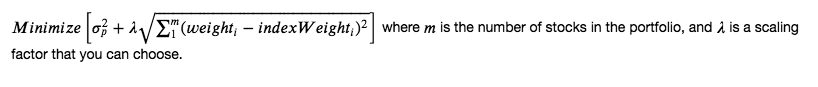## Install cvxpy and other libraries

import sys
!{sys.executable} -m pip install -r requirements.txt
Requirement already satisfied: colour==0.1.5 in /opt/conda/lib/python3.6/site-packages (from -r requirements.txt (line 1))
Collecting cvxpy==1.0.3 (from -r requirements.txt (line 2))

[33mYou are using pip version 9.0.1, however version 19.0.3 is available.
You should consider upgrading via the 'pip install --upgrade pip' command.[0m

## Imports

import cvxpy as cvx
import numpy as np
import quiz_tests_advanced

## What's our objective?

http://www.cvxpy.org/

Let's see how we can use optimization to meet a more advanced objective. We want to both minimize the portfolio variance and also want to closely track a market cap weighted index. In other words, we're trying to minimize the distance between the weights of our portfolio and the weights of the index.## Hints

### x vector

To create a vector of M variables
$$\mathbf{x} = \begin{bmatrix} x_1 &...& x_M \end{bmatrix}$$
we can use cvx.Variable(m)

### covariance matrix

If we have $m$ stock series, the covariance matrix is an $m \times m$ matrix containing the covariance between each pair of stocks. We can use numpy.cov to get the covariance. We give it a 2D array in which each row is a stock series, and each column is an observation at the same period of time.

The covariance matrix
$$\mathbf{P} = \begin{bmatrix} \sigma^2{1,1} & ... & \sigma^2{1,m} \ ... & ... & ...\ \sigma{m,1} & ... & \sigma^2{m,m} \ \end{bmatrix}$$

### portfolio variance

We can write the portfolio variance $\sigma^2_p = \mathbf{x^T} \mathbf{P} \mathbf{x}$

Recall that the $\mathbf{x^T} \mathbf{P} \mathbf{x}$ is called the quadratic form.
We can use the cvxpy function quad_form(x,P) to get the quadratic form.

### Distance from index weights

We want portfolio weights that track the index closely. So we want to minimize the distance between them.
Recall from the Pythagorean theorem that you can get the distance between two points in an x,y plane by adding the square of the x and y distances and taking the square root. Extending this to any number of dimensions is called the L2 norm. So: $\sqrt{\sum_{1}^{n}(weight_i - indexWeight_i)^2}$ Can also be written as $\left | \mathbf{x} - \mathbf{index} \right |_2$. There's a cvxpy function called norm()
norm(x, p=2, axis=None). The default is already set to find an L2 norm, so you would pass in one argument, which is the difference between your portfolio weights and the index weights.

### objective function

We want to minimize both the portfolio variance and the distance of the portfolio weights from the index weights.
We also want to choose a scale constant, which is $\lambda$ in the expression. This lets us choose how much priority we give to minimizing the difference from the index, relative to minimizing the variance of the portfolio. If you choose a higher value for scale ($\lambda$), do you think this gives more priority to minimizing the difference, or minimizing the variance?

We can find the objective function using cvxpy objective = cvx.Minimize(). Can you guess what to pass into this function?

### constraints

We can also define our constraints in a list. For example, you'd want the weights to sum to one. So $\sum_{1}^{n}x = 1$. You may also need to go long only, which means no shorting, so no negative weights. So $x_i >0$ for all $i$. you could save a variable as [x >= 0, sum(x) == 1], where x was created using cvx.Variable().

### optimization

So now that we have our objective function and constraints, we can solve for the values of $\mathbf{x}$.
cvxpy has the constructor Problem(objective, constraints), which returns a Problem object.

The Problem object has a function solve(), which returns the minimum of the solution. In this case, this is the minimum variance of the portfolio.

It also updates the vector $\mathbf{x}$.

We can check out the values of $x_A$ and $x_B$ that gave the minimum portfolio variance by using x.value

## Quiz

import cvxpy as cvx
import numpy as np

def optimize_portfolio(returns, index_weights, scale=.00001):
"""
Create a function that takes the return series of a set of stocks, the index weights,
and scaling factor. The function will minimize a combination of the portfolio variance
and the distance of its weights from the index weights.
The optimization will be constrained to be long only, and the weights should sum to one.

Parameters
----------
returns : numpy.ndarray
2D array containing stock return series in each row.

index_weights : numpy.ndarray
1D numpy array containing weights of the index.

scale : float
The scaling factor applied to the distance between portfolio and index weights

Returns
-------
x : np.ndarray
A numpy ndarray containing the weights of the stocks in the optimized portfolio
"""
# TODO: Use cvxpy to determine the weights on the assets
# that minimizes the combination of portfolio variance and distance from index weights

# number of stocks m is number of rows of returns, and also number of index weights
m = returns.shape

#covariance matrix of returns
cov = np.cov(returns)

# x variables (to be found with optimization)
x = cvx.Variable(m)

# euclidean distance (L2 norm) between portfolio and index weights
distance_to_index = cvx.norm(x - index_weights)

#objective function
objective = cvx.Minimize(portfolio_variance + scale * distance_to_index)

#constraints
constraints = [x >= 0, sum(x) == 1]

#use cvxpy to solve the objective
cvx.Problem(objective, constraints).solve()

#retrieve the weights of the optimized portfolio
x_values = x.value

return x_values

quiz_tests_advanced.test_optimize_portfolio(optimize_portfolio)
Tests Passed
"""Test with a 3 simulated stock return series"""
days_per_year = 252
years = 3
total_days = days_per_year * years

return_market = np.random.normal(loc=0.05, scale=0.3, size=days_per_year)
return_1 = np.random.uniform(low=-0.000001, high=.000001, size=days_per_year) + return_market
return_2 = np.random.uniform(low=-0.000001, high=.000001, size=days_per_year) + return_market
return_3 = np.random.uniform(low=-0.000001, high=.000001, size=days_per_year) + return_market
returns = np.array([return_1, return_2, return_3])

"""simulate index weights"""
index_weights = np.array([0.9,0.15,0.05])

print(f"The optimized weights are {x_values}, which sum to {sum(x_values):.2f}")
The optimized weights are [0.86739149 0.11639837 0.01621015], which sum to 1.00